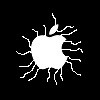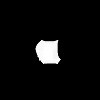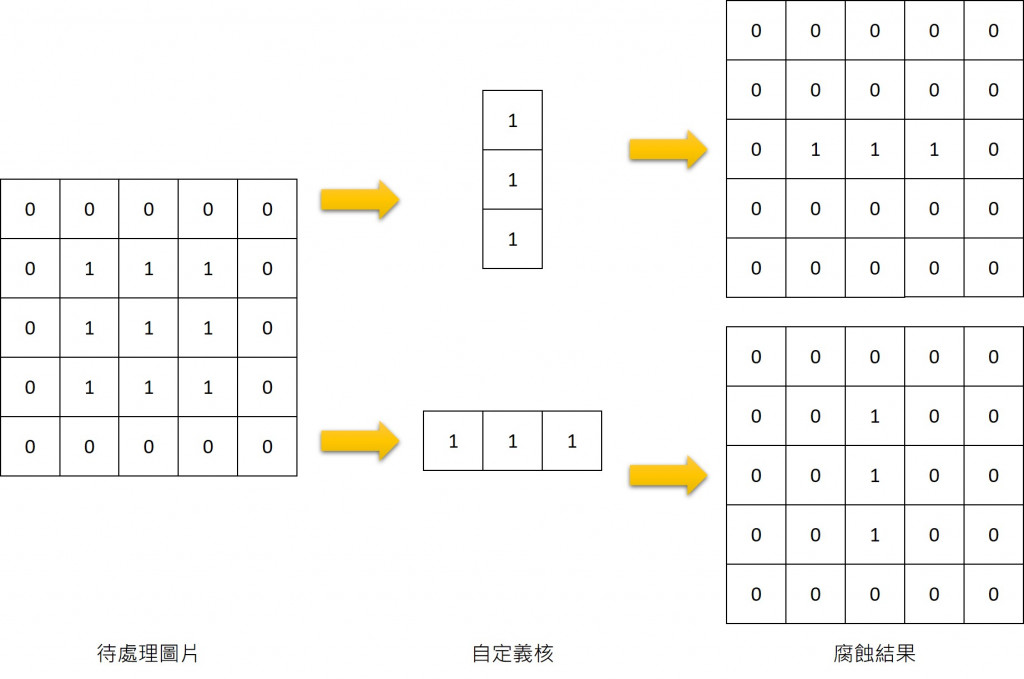#DAY 20
0

## 形態學操作－腐蝕、膨脹``````#image_morphology.py

import cv2 as cv
import numpy as np

img = cv.imread('apple2.jpg')
kernel = np.ones((3,3),dtype=np.uint8)
erosion = cv.erode(img,kernel)

cv.imwrite('./erosion.jpg',erosion)
````````````#image_morphology.py

import cv2 as cv
import numpy as np

img = cv.imread('apple2.jpg')
kernel = np.ones((6,6),dtype=np.uint8)
erosion = cv.erode(img,kernel)

cv.imwrite('./erosion.jpg',erosion)
````````````#image_morphology.py

import cv2 as cv
import numpy as np

img = cv.imread('apple2.jpg')
kernel = np.ones((4,4),dtype=np.uint8)
erosion = cv.erode(img,kernel)
dilation = cv.dilate(erosion,kernel)

cv.imwrite('./erosion.jpg',erosion)
cv.imwrite('./dilation.jpg',dilation)
``````

## 核結構(kernel)的概念1.anchor:調整核當中哪個對應的位置當作定錨點，預設為中心點(-1,-1)，
2.iterations:要幾次迭代的次數，預設為1

## 開運算、閉運算、梯度運算、禮帽運算與黑帽運算

``````cv2.morphologyEx(src,op,kernel)
``````

src是待處理圖片，中間的op所代表的是操作類別，kernel是自定義核結構，

OP類型 說明 處理過程
cv2.MORPH_ERODE 腐蝕 腐蝕(src)
cv2.MORPH_DILATE 膨脹 膨脹(src)
cv2.MORPH_OPEN 開運算 膨脹(腐蝕(src))
cv2.MORPH_CLOSE 閉運算 腐蝕(膨脹(src))
cv2.MORPH_GRADIENT 梯度運算 膨脹(src)-腐蝕(src)
cv2.MORPH_TOPHAT 禮帽運算 src-開運算(src)
cv2.MORPH_BLACKHAT 黑帽運算 close(src)-src``````import cv2 as cv
import numpy as np

#讀取待處理圖片
img = cv.imread('grape.jpg')
gray = cv.cvtColor(img,cv.COLOR_BGR2GRAY)
ret,binary = cv.threshold(gray,200,255,cv.THRESH_BINARY_INV)

#建立核結構
kernel = np.ones((8,8),dtype=np.uint8)

#開運算處理
opening = cv.morphologyEx(binary,cv.MORPH_OPEN,kernel)

#閉運算處理
closing = cv.morphologyEx(binary,cv.MORPH_CLOSE,kernel)

#梯度運算處理
gradient = cv.morphologyEx(binary,cv.MORPH_GRADIENT,kernel)

#禮帽運算處理
tophat = cv.morphologyEx(binary,cv.MORPH_TOPHAT,kernel)

#黑帽運算處理
blackhat = cv.morphologyEx(binary,cv.MORPH_BLACKHAT,kernel)

#禮帽+黑帽
twohat = cv.add(tophat,blackhat)

cv.imwrite('./binary.jpg',binary)
cv.imwrite('./opening.jpg',opening)
cv.imwrite('./closing.jpg',closing)
cv.imwrite('./gradient.jpg',gradient)
cv.imwrite('./tophat.jpg',tophat)
cv.imwrite('./blackhat.jpg',blackhat)
cv.imwrite('./twohat.jpg',twohat)

``````

## 內建核函數cv2.getStructuringElement()

``````cv2.getStructuringElement(shape,ksize[, anchor])
``````

cv2.MORPH_RECT 矩形結構類型，所有值為1
cv2.MORPH_CROSS 十字形結構類型，對角線數值為1
cv2.MORPH_ELLIPSE 橢圓形結構類型